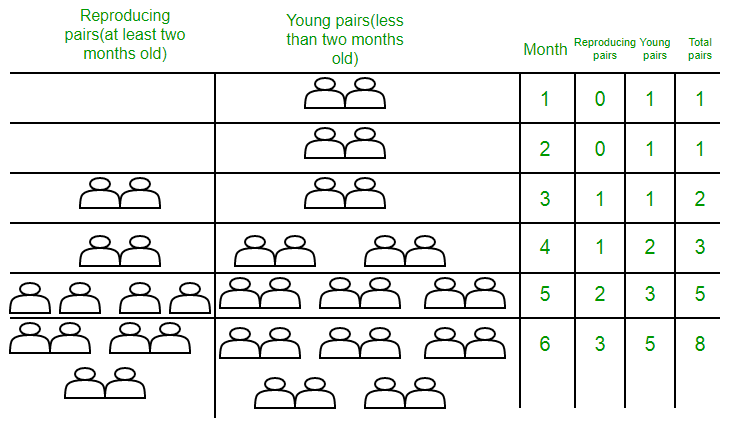# Puzzle 56 | Chinchillas Relations

• Difficulty Level : Medium
• Last Updated : 10 Feb, 2018

Q. A young pair of chinchillas (one of each sex) is placed on an island. A pair of chinchillas does not breed until they are 2 months old. After they are 2 months old, each pair of chinchillas produces another pair each month (See pic below). Find a recurrence relation for the number of pairs of chinchillas on the island after n months, assuming that no chinchillas ever die.Solution:

Let us denote the number of pairs of chinchillas after n months by f(n).

The chinchilla population can be modeled using a recurrence relation. At the end of the first month, the number of pairs of chinchillas on the island is f(1) = 1. Because this pair does not breed during the second month, f(2) = 1 also. To find the number of pairs after n months, add the number on the island the previous month, f(n−1) , and the number of newborn pairs, which equals f(n−2) , because each newborn pair comes from a pair at least 2 months old.

Consequently, the sequence {f(n)} satisfies the recurrence relation

f(n) = f(n−1) + f(n−2 )

for n ≥ 3 together with the initial conditions f(1) = 1 and f(2) = 1.This recurrence relation and the initial conditions uniquely determine this sequence.

If you closely observe, this is the recurrence relation for Fibonacci numbers. (See ‘Total Pairs’ column in the pic)

Hence, the number of pairs of chinchillas on the island after n months is given by the nth Fibonacci number.

Reference: http://www.maths.surrey.ac.uk/hosted-sites/R.Knott/Fibonacci/fibnat.html